## 2.10Fluid deformation

Conservation of momentum Eq. (2.19 ) includes the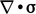term describing the forces within a ﬂuid. At a macroscopic level, the forces exist due to interactions between ﬂuid particles and change due to deformation of the ﬂuid as it moves.

From the velocity ﬁeld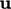, we need to isolate pure deformation of the ﬂuid from other characteristics of the ﬂow. The ﬁgure below shows a rectangular ﬂuid element under shear in the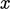-direction due to a uniform velocity gradient in the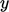-direction.

The velocity gradient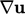calculates some rate of shear of the rectangular element. The upper diagram shows shear in one direction, known as parallel shear.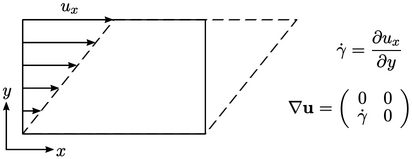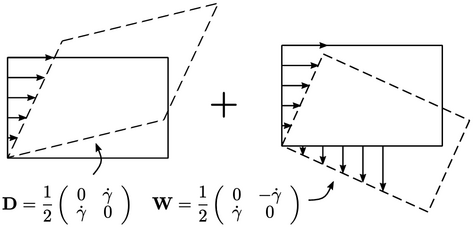Parallel shear can be decomposed into pure shear (left) and a rotation (right). The rotational component is represented by the skew part of, see Eq. (2.25 ). Since a rotation does not involve deformation, it must be removed from any measure of it, which leaves the symmetric part of thetensor.

Therefore, the rate of deformation tensor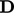and spin (rotational) tensor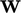are deﬁned as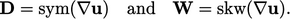(2.33)

### Trace of a tensor

The trace of a tensor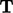is the sum of the diagonal components, producing a scalar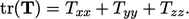(2.34)
Where the tensor is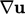, we conclude from Eq. (2.13 ) that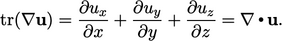(2.35)

### Deviatoric and spherical tensors

A tensor can be decomposed into the sum of its deviatoric part and a spherical part as follows: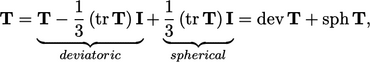(2.36)
where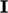is the identity tensor, see Eq. (2.30 ). The deviatoric part subtracts the mean of the trace from each diagonal component such that the resulting tensor is “trace-free”, i.e.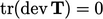.
Notes on CFD: General Principles - 2.10 Fluid deformation